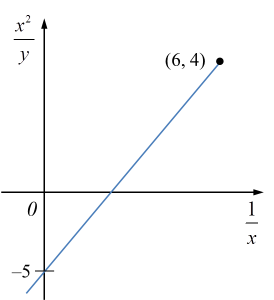\

# SPM Additional Mathematics 2018, Paper 1 (Question 11 – 13)

Question 12 (4 marks):
Diagram 5 shows a circle with centre O.Diagram 5

PR
and QR are tangents to the circle at points P and Q respectively. It is given that the length of minor arc PQ is 4 cm and
Express in terms of α,
(a) the radius, r, of the circle,
(b) the area, A, of the shaded region.

Solution:
(a)

(b)

Question 13 (3 marks):
Diagram 6 shows the graph of a straight lineDiagram 6

Based on Diagram 6, express y in terms of x.

Solution: